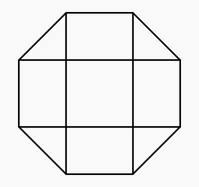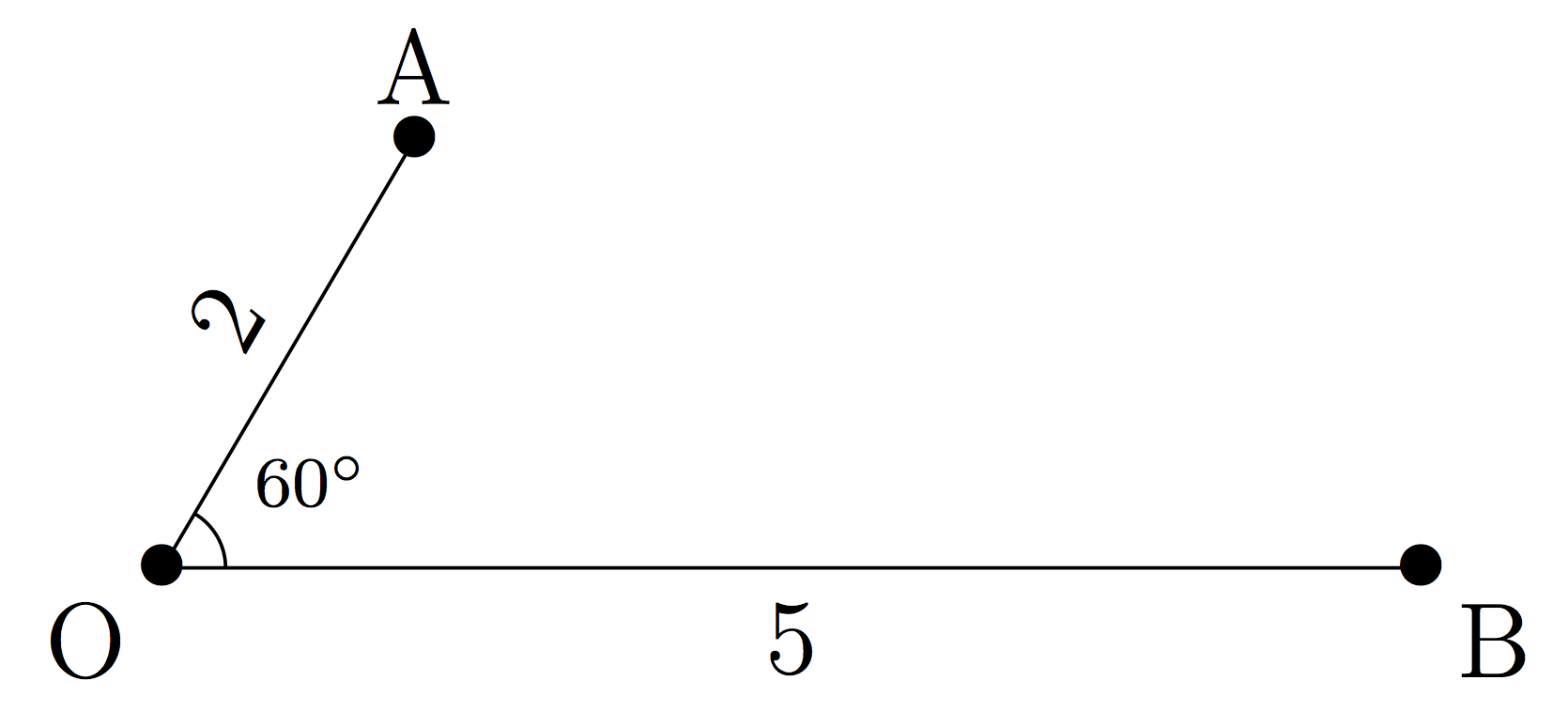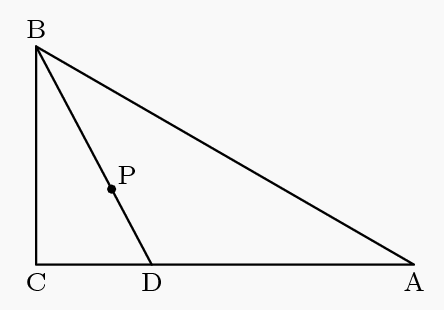###### back to index | new

Let $P(x) = x^2 - 3x - 9$. A real number $x$ is chosen at random from the interval $5 \le x \le 15$. The probability that $\lfloor\sqrt{P(x)}\rfloor = \sqrt{P(\lfloor x \rfloor)}$ is equal to $\frac{\sqrt{a} + \sqrt{b} + \sqrt{c} - d}{e}$ , where $a$, $b$, $c$, $d$, and $e$ are positive integers. Find $a + b + c + d + e$.

A point $P$ is chosen at random in the interior of a unit square $S$. Let $d(P)$ denote the distance from $P$ to the closest side of $S$. The probability that $\frac{1}{5}\le d(P)\le\frac{1}{3}$ is equal to $\frac{m}{n}$, where $m$ and $n$ are relatively prime positive integers. Find $m+n$.

Fifty tickets numbered with consecutive integers are in a jar. Two are drawn at random and without replacement. What is the probability that the absolute difference between the two numbers is $10$ or less?

Let $S$ be the set $\{1,2,3,...,19\}$. For $a,b \in S$, define $a \succ b$ to mean that either $0 < a - b \le 9$ or $b - a > 9$. How many ordered triples $(x,y,z)$ of elements of $S$ have the property that $x \succ y$, $y \succ z$, and $z \succ x$?

Two real numbers are selected independently and at random from the interval $[-20,10]$. What is the probability that the product of those numbers is greater than zero?

A region $S$ in the complex plane is defined by $S = \{x + iy: - 1\le x\le1, - 1\le y\le1\}.$ A complex number $z = x + iy$ is chosen uniformly at random from $S$. What is the probability that $\left(\frac34 + \frac34i\right)z$ is also in $S$?

Two circles of radius 1 are to be constructed as follows. The center of circle $A$ is chosen uniformly and at random from the line segment joining $(0,0)$ and $(2,0)$. The center of circle $B$ is chosen uniformly and at random, and independently of the first choice, from the line segment joining $(0,1)$ to $(2,1)$. What is the probability that circles $A$ and $B$ intersect?

Let $S$ be a square of side length $1$. Two points are chosen independently at random on the sides of $S$. Find the probability that the straight-line distance between the points is at least $\dfrac{1}{2}$.

Two concentric circles have radii $1$ and $2$. Two points on the outer circle are chosen independently and uniformly at random. What is the probability that the chord joining the two points intersects the inner circle?

Real numbers $x$, $y$, and $z$ are chosen independently and at random from the interval $[0,n]$ for some positive integer $n$. The probability that no two of $x$, $y$, and $z$ are within 1 unit of each other is greater than $\frac {1}{2}$. What is the smallest possible value of $n$?

Two points on the circumference of a circle of radius $r$ are selected independently and at random. From each point a chord of length $r$ is drawn in a clockwise direction. What is the probability that the two chords intersect?

Two real numbers are selected independently at random from the interval $[-20, 10]$. What is the probability that the product of those numbers is greater than zero?

A dart board is a regular octagon divided into regions as shown. Suppose that a dart thrown at the board is equally likely to land anywhere on the board. What is probability that the dart lands within the center square?Avi and Hari agree to meet at their favorite restaurant between 5:00 p.m. and 6:00 p.m. They have agreed that the person who arrives first will wait for the other only 15 minutes before leaving. What is the probability that the two of them will actually meet at the restaurant, assuming that the arrival times are random within the hour? Express your answer as a common fraction.

Let $a$ and $b$ be two randomly selected points on a line segment of unit length. What is the probability that their distance is not more than $\frac{1}{2}$?

Randomly select $3$ real numbers $x$, $y$, and $z$ between 0 and 1. What is the probability that $x^2 + y^2 + z^2 > 1$?

There are several equally spaced parallel lines on a table. The distance between two adjacent lines is $2a$. On the table, toss a coin with a radius of $r$, $(r < a)$. Find the probability that the coin does not touch any line.

Joe breaks a $10$-meter long stick into three shorter sticks. Find the probability that these three sticks can form a triangle.

Break a stick into two parts. What is the probability that the length of one part is at least twice of that of the other?

Two people agree to meet at a place some time in the next 10 days. They have also agreed whoever arrives the place should wait for the other for 3 days and then leave. What is the probability that they will see each other?

In the following diagram, $\overline{AO}= 2$, $\overline{BO} = 5$, and $\angle{AOB} = 60^\circ$. Point $C$ is selected on $\overline{BO}$ randomly. Find the probability that $\triangle{AOC}$ is an acute triangle.A girl and a guy are going to arrive at a train station. If they arrive within $10$ minutes of each other, they will instantly fall in love and live happily ever after. But after $10$ minutes, whichever one arrives first will fall asleep and they will be forever alone. The girl will arrive between $8$ AM and $9$ AM with equal probability. The guy will arrive between $7$ AM and $8:30$ AM, also with equal probability. Find the probability that the probability that they fall in love.

Triangle $ABC$ is a right triangle with $\angle ACB$ as its right angle, $m\angle ABC = 60^\circ$ , and $AB = 10$. Let $P$ be randomly chosen inside $ABC$ , and extend $\overline{BP}$ to meet $\overline{AC}$ at $D$. What is the probability that $BD > 5\sqrt2$?Three numbers in the interval $\left[0,1\right]$ are chosen independently and at random. What is the probability that the chosen numbers are the side lengths of a triangle with positive area?

A point is chosen at random within the square in the coordinate plane whose vertices are $(0, 0), (2020, 0), (2020, 2020),$ and $(0, 2020)$. The probability that the point is within $d$ units of a lattice point is $\tfrac{1}{2}$. (A point $(x, y)$ is a lattice point if $x$ and $y$ are both integers.) What is $d$ to the nearest tenth?